Proof that the golden ratio is irrational by contradiction

What is the golden ratio is good explained in  Wikipedia.org/wiki/Golden_ratio

Summary: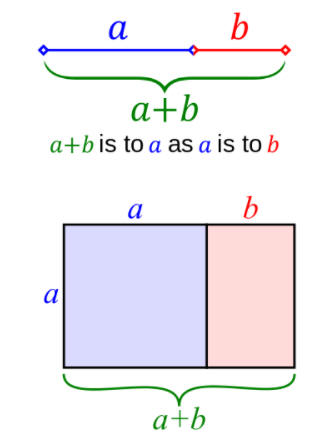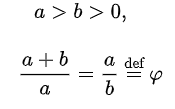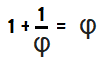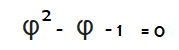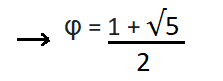Let´s assume that φ is rational.

a/b = φ is completely reduced, so its greatest common divisor is 1   (  gcd(a,b) = 1  )

For example, 4/8 can be reduced to 1/2

b < a  (from definition)

a/b = (1+ √5)/2  < ( 1 + √9) / 2 = 2   →   a < 2 b

a/b =  = (1+ √5)/2) > (1+ √4)/2 = 3/2  → a > 3/2 b  (not necessary..)

a < 2 b  →  a - b < b

From  1/φ = φ - 1

b/a = a/b -1 = (a - b)/b

→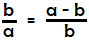,  with a-b < b and with b < a

b/a is completely reduced, but is equal to another fraction with both a smaller denominator and a smaller  numerator.   (For example,  3/4 = 2/3)

This is a contradiction, so φ is not rational.

I hope this  is useful for somebody. Anyway it is for myself, to refresh my memory sometimes in the future

Rinze Joustra